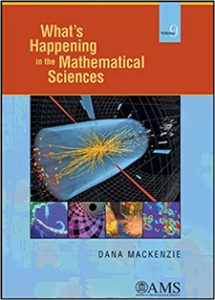Select Page

## WHIMS Volume 9What’s Happening in the Mathematical Sciences, Volume 9 covers the years from 2010 to 2012. Below is an annotated table of contents.

1. Massive Breakthrough.

In 2012, physicists at CERN completed the search for the last subatomic particle in the Standard Model of quantum physics, the Higgs particle. As the culmination of a multi-decade \$9 billion project, this discovery was emblematic of today’s “big science.” And yet few people realize that it all began with a few equations written on one sheet of A4 paper. This article explains the chain of ideas that led from the “Higgs symmetry-breaking mechanism” (which is pure mathematics) to the “Higgs field” (which gives all other particles their mass) to the Higgs particle.

Branch of mathematics: Mathematical physics, analysis, statistics.

2. Tubing through Hyperspace.

Also in 2012, three separate but related open problems were solved concerning the geometry of tori (or “inner tubes”). The Willmore Conjecture asked for the shape of tori with the smallest “bending energy,” and the Lawson and Pinkall/Sterling conjectures had to do with shapes of zero mean curvature (i.e., soap-film-like) or constant mean curvature (soap-bubble-like) tori in a hypersphere. Yes, in a hypersphere you can blow a bubble with the shape of an inner tube!

Branch of mathematics: Geometry, differential equations.

3. Tsunamis: Learning from Math, Learning from the Past.

Fifteen thousand people died in the 2011 Japanese tsunami, even though Japan is the best-prepared nation on Earth. How did it happen? This article explores the mathematics behind tsunamis, from the simplest shallow-water equations to the complicated models that are used to issue tsunami warnings and to predict the effects of future tsunamis. These models show that Japanese planners should have paid better attention to ancient history.

Branch of mathematics: Differential equations, applied mathematics.

4. Today’s Forecast: Ten Percent Chance of Burglary.

Earthquakes and crime have two things in common: they do not occur with uniform frequency everywhere, and they both have a “self-exciting” property in which shocks (or crimes) are followed by aftershocks (or copycat crimes). Mathematical equations exploit this similarity to predict where crimes are more likely to happen next. This “predictive policing” has led to more than 10 percent drops in burglaries in cities like Los Angeles and Santa Cruz.

Branch of mathematics: Statistics, applied mathematics.

5. Topologists Cross Four off “Bucket List.”

The subject of three-dimensional topology is like a continent with eight countries. At one end of the continent, positively curved manifolds (i.e., curved spaces) are well understood thanks to Grigory Perelman’s proof of the Poincare Conjecture in 2006. At the opposite end of the continent lies the land of hyperbolic manifolds, where the wild things live. Until recently the variety of hyperbolic manifolds seemed indescribable, but now, thanks to the Virtual Haken Conjecture (which had been seen as probably true) and the Virtual Fibering Conjecture (which had been seen as probably false — right until the week before it was proved) have now brought some tameness even to the wildest jungle of three-dimensional space.

Branch of mathematics: Geometric topology, group theory.

6. Mathematicians Do the Twist.

Ever since it was invented, the Rubik’s cube has been the mathematician’s favorite puzzle, as probably the most complicated example of a finite group that most people ever encounter. This chapter tells about three recent advances in Rubik’s cube math. First is the proof that God can solve any Rubik’s cube in 20 moves. Second is an estimate of “God’s number” for arbitrarily large (n-by-n-by-n) Rubik’s cubes. And finally, robots break the 5-second barrier in physically solving a cube — and humans, amazingly, are not far behind.

Branch of mathematics: Computational mathematics, group theory.

7. The Right Epidemic at the Right Time.

The influenza pandemic of 2009, which started in Mexico, was the first global flu scare of the 21st century. It ended up being less severe than expected, although “less severe” is relative when talking about a disease that killed thousands of people. The pandemic was the first one that could be fully tracked and predicted by mathematical models. The models were hobbled by inaccurate data; the production of vaccines was a fiasco; but lessons were learned that should allow mathematical modelers and public-health officials to work together more effectively in the future.

Branch of mathematics: Mathematical biology, applied mathematics.

8. Thinking Topically.

Topic modeling is the development of algorithms to teach a computer to figure out what topics are covered in a large corpus of documents. A breakthrough called latent Dirichlet allocation breaks down the barriers between math and the humanities, allowing truly empirical, quantitative analysis of large bodies of literature. Applications include algorithms to automatically identify signs of cyber-bullying on a social-media website.

Branch of mathematics: Probability, statistics, applied mathematics.

9. Thinking Tropically.

Tropical geometry is a bizarre form of geometry in which curves are replaced by stick figures, and the familiar operations of addition and multiplication are replaced with “minimization” and addition. Strangely, many of the deep theorems and problems of algebraic geometry (the study of curves or surfaces defined by algebraic equations) have exact analogues in tropical geometry, suggesting that the latter can be used to give us information about the former.

Branch of mathematics: Algebraic geometry.

Cover images: Computer model of collision forming Higgs particles; locations of pre-computed earthquakes used in tsunami model; a Willmore surface; Rubik’s cube solver’s hands are faster than the eye (and the camera); predictive policing model.